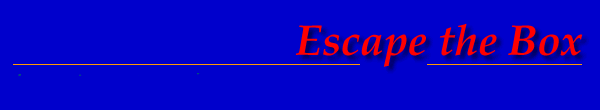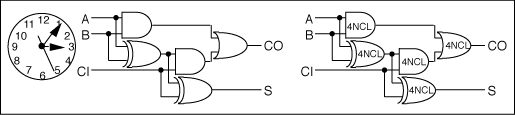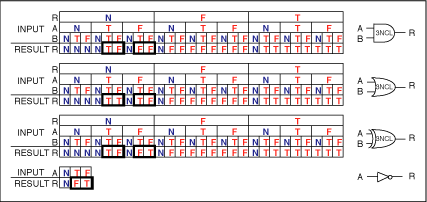Theseus Research : Technical Papers : Considerations of Completeness Page 5 of 11

The Universality of the NULL Function

Gates vary in their behavior only for the data function. The NULL function is identical for all gates. Any arbitrary combination of gates will behave identically with the NULL wavefront. They will all transition to NULL. So the NULL wavefront behavior can be ignored in the specification of a combinational expression. Only the behavior of the data wavefront need be considered when specifying a combinational expression.

Completeness of Participation

If there is a don't care signal anywhere in the expression, one which may or may not transition, it would not be possible to logically determine when a resolution is complete. So every gate and every signal path of the combinational expression effectively participates in every wavefront resolution. This will be referred to as completeness of participation.

Summary of 4NCL Completenesses

There are a number of completeness relationships that contribute to the logical determination of 4NCL.

Completeness of transition between disjoint logical value domains.

Completeness criterion.

Completeness of participation.

Single-form completeness of expression

Relationship of 4NCL to Boolean Logic

Since 4NCL uses TRUE and FALSE to differentiate data values, 4NCL can be directly mapped gate for gate with a Boolean logic expression to form a 4NCL combinational expression as shown in Figure 6.

Figure 6. Boolean logic full adder and the corresponding 4NCL full adder.4NCL as a Standard of Reference

4NCL can be used as a standard of logical completeness for combinational expression. As an expression deviates from 4NCL logical completeness, the deviations can be characterized as compromises of the completeness relationships. In this way it is possible to see more clearly the inherent nature of other forms of expression and to relate them to each other.

In this instance the consequences of reducing the number of logic values will be considered.

Can a 3 value logic be logically complete?

The four value logic can be reduced to a three value logic that expresses the completeness criterion by replacing the expression of the INTERMEDIATE value with a hysteresis behavior of the gate as shown in Figure 7. This forms a three value NULL Convention Logic or 3NCL.

Figure 7. Correspondence of 4NCL and 3NCL.

 4NCL 3NCL TRUE TRUE FALSE FALSE INTERMEDIATE Hysteresis Behavior NULL NULL

In 4NCL, the INTERMEDIATE value indicates a partial completion state to be ignored by the watcher. The partial completion states can be effectively ignored with a hysteresis behavior or state holding behavior of each gate. When the input of a gate is completely data, the gate outputs a data value and maintains the data value until the input of the gate is completely NULL, whereupon, the gate outputs a NULL value and maintains the NULL value until the input of the gate is completely data, and so on. Instead of being explicitly expressed as a logic value at the gate output, the partially complete states are ignored by the gate output. Only wavefronts of NULL values and data values flow through the gates and through the expression.

This hysteresis behavior can be expressed logically as a feedback relationship making the gate a state machine. The truth tables expressing the hysteresis behavior for the 3NCL AND gate, OR gate, XOR gate and NOT gate are shown in Figure 8. The NOT gate is a single input gate that cannot posses any intermediate input states so it does not require hysteresis to explicitly express completeness for both data and NULL. The input R value is the output result value fed back to the input. The gate data function is expressed by the values inside the bold boxes. This feedback path includes a time relationship that must be expressed to completely characterize the behavior of a combinational expression.

Figure 8. 3NCL gates.